## Monday, April 26, 2010

### Electromagnetism

Electromagnetism is the science of the properties and relationship between electric currents and magnetism.
An electric current creates a magnetic ﬁeld and a moving magnetic ﬁeld will create a ﬂow of charge.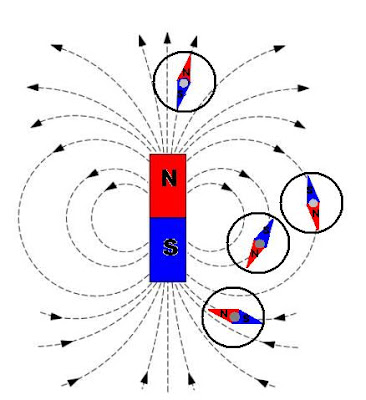A bar magnet produces magnetic fields the around it.
Magnetic fields also can be produce by an electric current in a wire.If you hold a compass near a wire through which current is ﬂowing, the needle on the compass will be deﬂected.

Electromagnet is a temporary magnet. It is made by winding a coil of insulated wire round a soft iron core.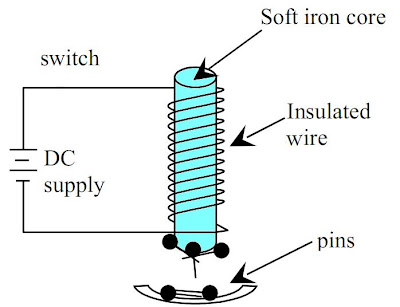The magnetic ﬁeld produced by an electric current is always oriented perpendicular to the
direction of the current ﬂow. When we are drawing directions of magnetic ﬁelds and currents,
we use the symbol ⊙ and .

The symbol⊙ for an arrow that is coming out of the page and
the symbol for an arrow that is going into the page.

It is easy to remember the meanings of the symbols if you think of an arrow with a head and a
tail.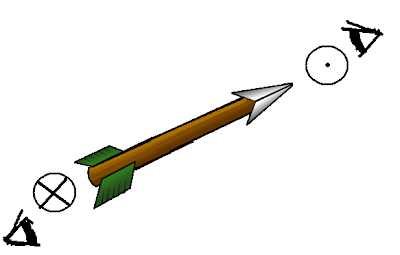When the arrow is coming out of the page, you see the head of the arrow (⊙).
When the arrow is going into the page, you see the tail of the arrow ().

There is a simple method of showing the relationship between the direction of the current ﬂowing in a conductor and the direction of the magnetic ﬁeld around the same conductor. The
method is called the Right Hand Rule.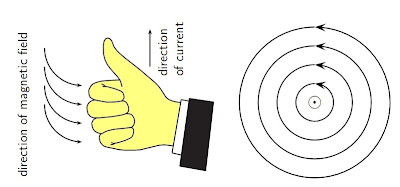## Saturday, April 24, 2010

### Power label of appliancesA halogen bulb labeled 240 V, 50 W:

~ When connected to a 240 V voltage source, the bulb will shine with normal intensity. Power dissipated is 50 W

~ When connected to a voltage less than 240 V, the bulb will shine with less intensity and power is less than 50 W

~ When connected to a voltage more than 240 V, the bulb will shine with brighter intensity and power is more than 50 W. Life span of the light bulb will be shortened and the light bulb is burned out .

Find out why Energy Star compliant products use less electricity while helping stop global warming and save money?## Saturday, April 10, 2010

### Puthandu( Tamil New Year )

Puthaandu Nalvazhthukkal to all my Tamil friend.

### Superconductors

There is a special type of conductor, called a superconductor that has no resistance, but the materials that make up superconductors only start superconducting at critical temperatures (very low temperatures approximately -170◦C).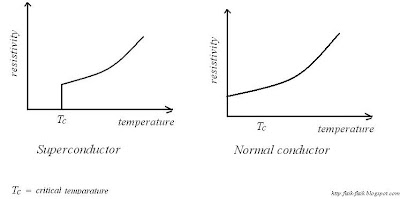Best conductors like silver, copper and gold do not show superconductivity.

Special properties:

• Allows the flow of electric current with minimal loss ofenergy
• Negates any applied external magnetic fields
Superconductors are used in transportation, electronic components, energy storage, power cables, etc.

## Wednesday, April 7, 2010

### PEKA - Form 4 - PENDULUM

Aim of the experiment:
To investigate how the period of a simple pendulum varies with its length.

Hypothesis:
The longer the length of a simple pendulum, the longer the period of oscillation.

Variables:
Manipulated: The length of the pendulum, l
Responding: The period of the pendulum, T
Constant: The mass of the pendulum bob, gravitational acceleration

Apparatus/Materials:
Pendulum bob, length of thread about 100 cm long, retort stand, stopwatch

Setup:Procedure:

1. The thread is tied to the pendulum bob. The other end of the thread is tied around the arm of the retort stand so that it can swing freely. The length of the pendulum, l is measured to 80 cm as per the diagram.
2. With the thread taut and the bob at rest, the bob is lifted at a small amplitude (of not more than 10°). Ensure that the pendulum swings in a single plane.
3. The time for ten complete oscillations of the pendulum is measured using the stopwatch.
4. Step 3 is repeated, and the average of both readings are calculated.
5. The period of oscillation, T is calculated using the average reading divided by the number of oscillations, i.e. 10.
6. T2 is calculated by squaring the value of T.
7. Steps 1 to 6 are repeated using l = 70 cm, 60 cm, 50 cm, and 40 cm.
8. A graph T2 versus l is plotted.
Data:Analysis :Discussion:
The graph of T2 versus l shows a straight line passing through the origin. This means that the period of oscillation increases with the length of the pendulum, with T2 directly proportional to l.

Conclusion:
The longer the length of the pendulum, the longer the period of oscillation. The hypothesis is proven valid.

Precaution :
The experiment was carried out in an enclosed room to avoid the influence of wind.

## Monday, April 5, 2010

### PEKA - Electromotive force and Internal Resistance

Aim :

To study the relationship between the potential difference and current of the dry cells

Hypothesis:

The potential difference across the terminals of dry cells decrease when the current supplied by the cells increase .

Variables:

Manipulated: Current, I
Responding: Potential difference across the terminals, V
Constant: Dry cell used

Apparatus/Materials:

Dry cells, ammeter, voltmeter, rheostat, resistor, switch, connecting wires

Procedure:1. A circuit as shown in the diagram above is set up.
2. The switch is started. The rheostat is adjusted until the ammeter reading shows 0.2 A. The voltmeter reading is recorded.
3. Step 2 is repeated with current values of 0.3 A, 0.4 A, and 0.5 A.
4. A graph of V against I is plotted. The slope of the graph is calculated and the V-crossing is extrapolated.

Results: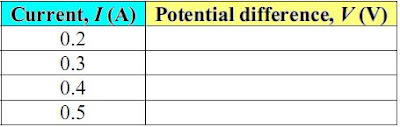Analysis:
Internal resistance, r = slope value
Electromotive force, E = V-crossing

Conclusion:
The hypothesis is accepted, from the experiment, the potential difference across the dry cells when the current supplied increases.

## Sunday, April 4, 2010

### Power rating and energy consumption

You should be able to :
1. compare power rating and energy consumption of various electrical appliances.
2. compare various electrical appliances in term of efficient use of energy.
3. suggest ways of increasing energy efficiency.

Notes: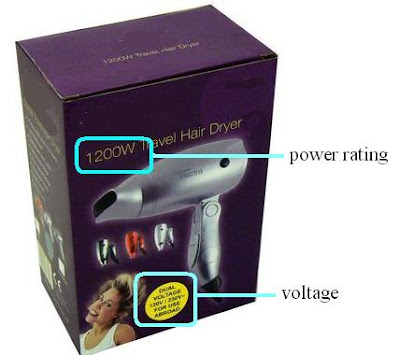Most electrical appliances indicate their voltage and power ratings on their label.
The label "120V 1200W" means that the hair dryer will consumed 1200J of electrical energy every second if it is connected to the 12o V main supply.

Electrical energy consumed , E = power rating, P x Time of usage, t

The energy consumption of an electrical appliance depends on the power rating and time of usage.
• the larger the power rating in the electrical appliance, the more energy is used in every second.
• the longer the usage time, the more electrical energy is consumed.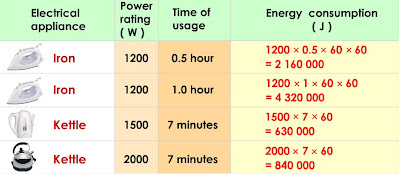The efficiency of an electrical appliance is determined by the ratio of used output to the input energy.

Efficiency = (Useful power output / power input ) x 100%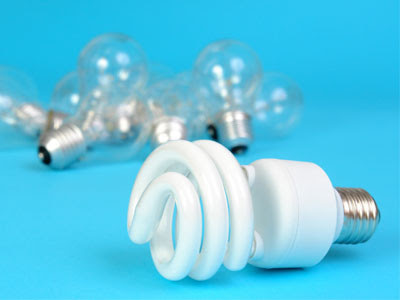source: http://science.howstuffworks.com/earth/green-technology/sustainable/home/cfl-bulb.htm

A tungsten-filament light bulb uses electrical energy to heat up the filament to a hight temperature until the tungsten atoms are excited before they emit a useful amount of visible light. However, most of the energy is lost to the surrounding in form of heat energy.

The fluorescent bulb activates phosphor coating inside the lamp gives off light whrn it is exposed to ultraviolet radiation. the bulb doesn't use heat to create light, which is more energy efficient.

Ways to reduce energy wastage:

i) Scheduled maintenance enable an electrical appliances to function efficiency in ensuring the efficiency of electrical energy usage.
ii) Buy high energy product.

## Thursday, April 1, 2010

### Current-carrying coil in a MAgnetic FieldA moving- coil meter ( Ammeter) can be used to measure direct current.
The coil is pivoted on a bearing and its rotation is resisted by hair springs which acts as control springs above and below it.The soft iron cylinder at the center of the coil is fixed and together with the curved poles of the magnet, it produces a radial field.
When current flows through the coil, an electric field is created as a result of the interaction between the two magnetic fields. this produces a pair of turning forces acting on the coil. The turning forces cause the coil to rotate until stopped by an equal and opposing force on the coil by the springs.
The greater the current, the greater the direction shown by the pointer attached to the coil. hence meter has a linear scale.

The sensitivity of the moving-coil meter is increased by having
i. more number of turns in the coil,
ii. stronger magnets
iii. weaker control springs,
iv. a greater number of turns in the coil.